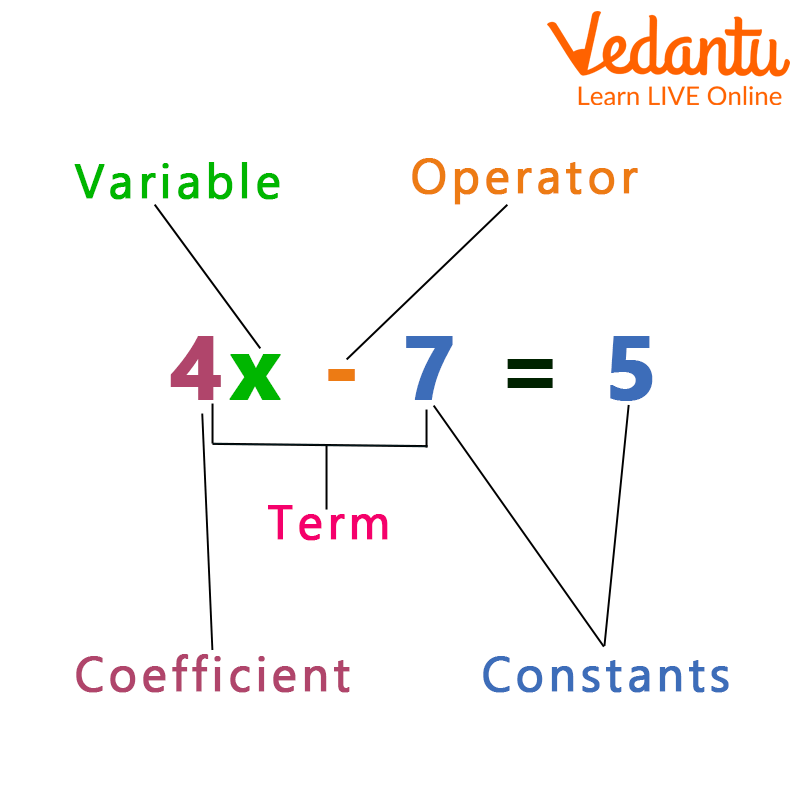Courses
Courses for Kids
Free study material
Free LIVE classes
More

# Coefficient ExamplesLIVE
Join Vedantu’s FREE Mastercalss

## Introduction:

A variable of a single term or the terms of a polynomial is multiplied by a number or an alphabet to produce a coefficient. For instance, the coefficient in the expression ${\rm{7x}}$ is 7. In the expression ${\rm{3xy}}$, x's coefficient is ${\rm{3y}}$.

### What is a Coefficient in Math?

A coefficient in mathematics is a value that is multiplied by the variable in a single term or the terms of a polynomial. It can be a number or any symbol that represents a constant value. It is typically a number, however in other expressions a letter might be used instead. In the formula: ${\rm{a}}{{\rm{x}}^{\rm{2}}} + {\rm{bx}} + {\rm{c}}$, for instance, x is the variable and a and b are the coefficients.Coefficient in an Equation

### Numerical Coefficient with Example:

A set amount multiplied by a variable is what is referred to as a numerical coefficient. Take into account the supplied monomial ${\rm{7abc}}$. 7 is the result for the coefficient of multiplying the fixed integer by the variables ${\rm{abc}}$. Therefore, the word "${\rm{7abc}}$" has a numerical coefficient of "7."

### Coefficient in Algebra:

A multiplier that multiplies two variables.

As an illustration, ${\rm{6z}}$ stands for 6 times $z$. Since z is a variable, 6 is a coefficient.

For variables without a value, the coefficient is 1.

For instance, ${\rm{x}}$ is really ${\rm{1x}}$.

### Coefficient of a Term

Follow the instructions below to determine a term's coefficient for a variable:

Step 1: Enclose the variable and its corresponding power, whose coefficient we are determining.

Step 2: Ignore that variable and take into account any other numbers or variables it was used with. The coefficient will then be that.Coefficient in an Equation

### Tips and Tricks on Coefficient:

• When using coefficients, keep the following things in mind.

• A variable always has a coefficient associated with it.

• If a variable's coefficient is 1, then it has no associated value.

### Conclusion

In mathematics, a coefficient is the multiplicative factor of an expression, a polynomial term, or a series term. Although it might be any sentence, it typically appears as a number. If the coefficients are variables in and of themselves, they may also be known as parameters.

### Coefficient Examples:

Example 1: Find the coefficient of the term $x^2$ in the formula $a{x^2} + bx + c$.

Ans: In the statement above, we can see that with ${{x^2}}$ a is written, thus ‘a’ will be its coefficient.

Example 2: Find the numbers that make up the coefficients in the algebraic statement $3{{\rm{x}}^2} - 2{\rm{y}} + 5$.

Ans: There are three terms in the provided expression. The numerical coefficient in the expression ${\rm{3}}{{\rm{x}}^{\rm{2}}}$is 3, the coefficient in the expression $- 2{\rm{y}}$ is 2, and 5 is a constant.

Therefore, 3 and -2 are the numerical coefficients.

Last updated date: 25th Sep 2023
Total views: 81.6k
Views today: 0.81k

## FAQs on Coefficient Examples

1. What is Coefficient in algebra?

A coefficient in mathematics is a value that is multiplied by the variable in a single term or the terms of a polynomial.

2. Determine the coefficient of $- 4{\rm{x}}$?

The coefficient of $- 4{\rm{x}}$ is $- 4$.

3. Is coefficient always a number?

A coefficient may not always be a number.

A coefficient is a multiplicative factor in a polynomial, series, or expression in mathematics.They may also be referred to as parameters if the coefficients are variables in and of themselves.

4. What do the terms in the expression mean?

Term constructions make up an algebraic expression. A term can be a constant, a variable, or a set of related variables.

5. Determine the coefficient of  ${{\rm{x}}^{\rm{2}}}$ in the equation: ${x^2} - 3{\rm{x + 5 = 0}}$

The coefficient of  ${{\rm{x}}^{\rm{2}}}$ in the equation: ${x^2} - 3{\rm{x + 5 = 0}}$ is 1.